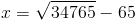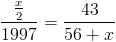August 6, 2010

Rendering Equations in GMail and Elsewhere

I just found out this workaround for rendering equations in GMail and it was so awesome that I felt I had to share it. Even if you don't use GMail, you might find this useful, if you ever have to work with equations online, because I'm going to show you 2 tools that help you with mathematical equations.

First, the "problem."

Have you ever wanted to send someone an email with an equation? This happened to me today when I wanted to send Dr. McCabe some math I was working on for a problem he threw at us on Wednesday. He likes to pop by the office and give us math problems to keep us on our toes. I'll give you guys the math problem when I find out for certain that I have the description correct.

Anyhow, I wanted to add a couple of equations to the email, but I wanted them to look nice. Let's say these were the equations:

x=sqrt(34765)-65

(x/2)/1997 = 43/56+x

It's much nicer if they look like this:Lots of word processors have equation editors, but GMail does not. There is a cool workaround that's also very easy to use.

You have to enable the ability to insert images into your GMail messages (it's a Google Labs feature) and then, instead of inserting an image from your hard drive, you choose to insert an image from a url. Here's the cool part: there's a website that will generate an equation image from a URL. It's called http://texify.com

If you type your equation using LaTeX syntax in place of the angle brackets in the following url, you'll get the rendered equation:

http://www.texify.com/img/<>.gif

The cool part is that if you type the above first and then add the equation, GMail gives you a live preview as you're typing.

The first equation looks like this in LaTeX: x=\sqrt{34765}-65

My web editor messes up the link, but you can take this URL:

http://www.texify.com/img/x=\sqrt{34765}-65.gif

...and paste that in your address bar and you'll see that it renders the equation. Neat! For documentation on how texify works, check out http://www.texify.com/.

But what if you don't know LaTeX? There are some good online editors that will generate the LaTeX for you, and show you what the syntax is. This is a good one.

Even better, if you don't use GMail, that equation editor will render the image for you and allow you to download a gif and paste it into any application. So, just for fun, you should go send some mathematical equations and expressions to people.

Posted by James at August 6, 2010 11:09 AM